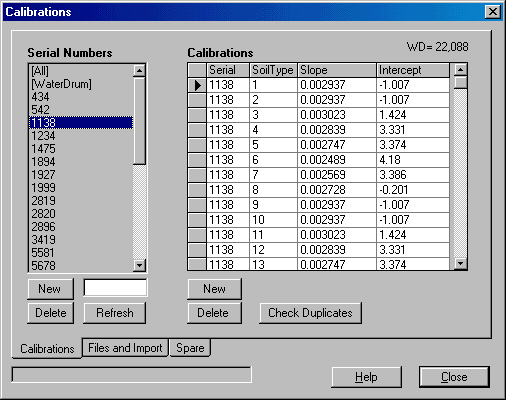# Calibrations

All data from neutron probes are saved as raw counts, and converted to volumetric soil mositure using calibration equations.#### Serial number method

The volumetric soil moisture (VSW) is calculated from...

` VSW = Count * Slope + Intercept`

There is no limit to the number of equations, and they can be different for each neutron probe. Each equation is for a different soil type, and a separate equation can be allocated to every depth at each tube.

It has been found in practice that differences between neutron probes can be compensated by taking a standard count in a water drum, and it is recommended that every neutron probe is regularly tested by taking a count in a water drum.

For routine commercial use, the most reliable and accurate method is to use the same equation at every depth - in this way the user can clearly see the water use from diffrent depths in the profile without having to correct for different soil textures. However, Probe for Windows allows you to use a separate equation at every depth.

#### Water drum ratio method

A more flexible way of using calibration equations is the water drum ratio method.

`VSW = (Count/WaterDrumCount) * Slope + Intercept`

The standard water drum count is posted instead of the probe serial number, and new calibrations do not need calculating and entering if the standard count ever changed. Prwin uses Serial Number = 1 for the 'water drum ratio' equations.

Users should be very carefull if they change the standard count or equations. The CPN 503DR is so stable that if the water drum count ever changes a fault is indicated and the probe should be serviced and checked.

After the unit is returned from servicing a standard count in the water drum should be taken. Only if the count is significantly different (change > 100) should it be changed in the 503 DR. If the 'serial number' equation method is being used a new serial number should be entered into the neutron ptobe and new calibration equations calculated and entered into the software.

See here for more details on user checking and calibration of neutron probes.